# Seaborn Histogram

You can easily create and style a histogram in Seaborn with just a few steps. Let’s get started. You will need a few dependencies to ensure that the plot is shown.  The dependencies that you essentially need to load are Matplotlib and Seaborn. However, let’s load the standards such as Pandas and Numpy also in case there is a need to change the data set to use the Seaborn histogram. For this example, I am using the NBA players dataset that you can find on Kaggle.com. I am interested in the height distribution from 1950 to 2018. Visualize Data with Python. Interpret Large Datasets. A/B Test Your Hypothesis at CodeAcademy!import pandas as pdimport numpy as npimport matplotlib.pyplot as pltimport seaborn as sns

Next, let’s access the Seaborn histograms which is  produced by the attribute distplot

Histograms are used to evaluate how your data is distributed. So to begin the tutorial lets take a look at the normap distribution using a array or list of data.

Lets Generate a distrubution of Data using Numpy

x = np.random.normal(size=100)

Now to generate a historgram, we only need the histogram function in Seaborn we can initiate the function using displot()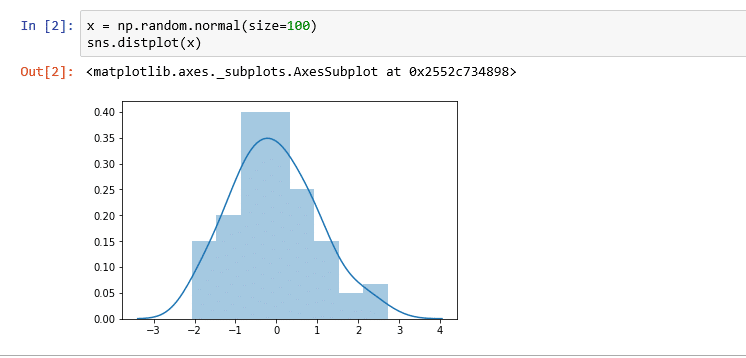This data is easy to read due to its normal distrubution. However, let’s take a look at some data that is not in a exact normal distribution.You ca

Changing the Color of the Histogram

You can easily change the color of your histogram by adding an argument color to the inside of the function.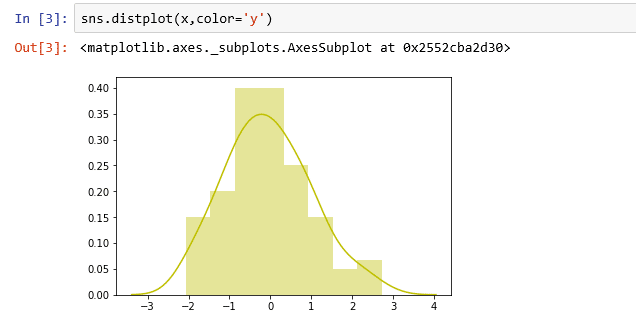The rug parameter in the displot() function will allow you to see the indicator of density in your graph. The rug parameter takes a boolean value.

sns.displot(x,color=’r’,rug=True)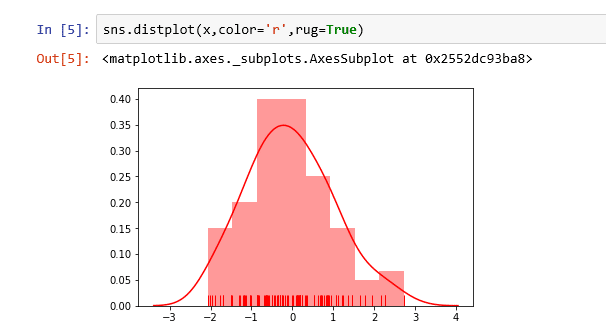We are using the Players dataset to see a distrubution of height.  Once initiating the histogram, you can easily see that this reflects real life data. But we can see that majority of the vales are distributed normally.

sns.distplot(df[‘height’])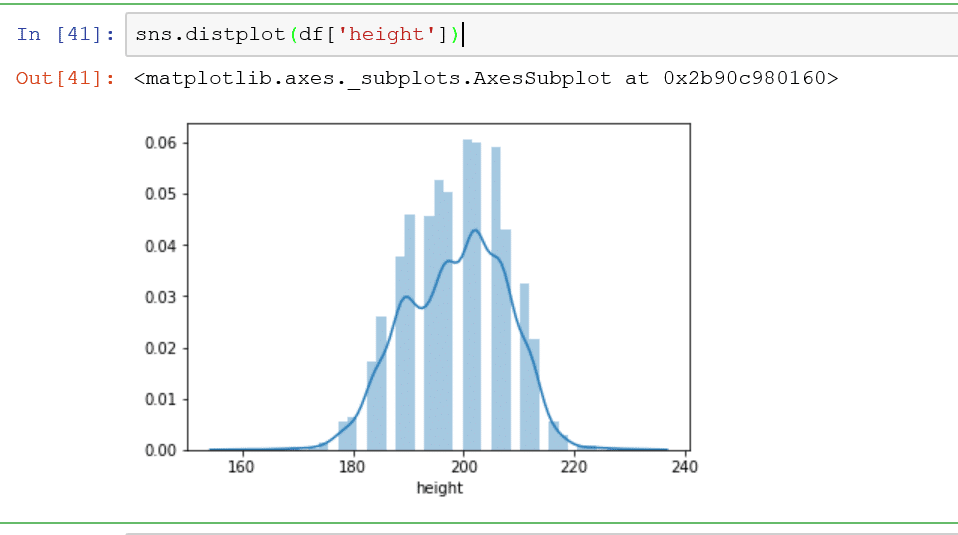Changing the number of bins in your histogram

This is the default histogram plot that has the default bins. You can also customize the number of bins using the bins parameter in your function.

sns.distplot(df[‘height’], bins=20)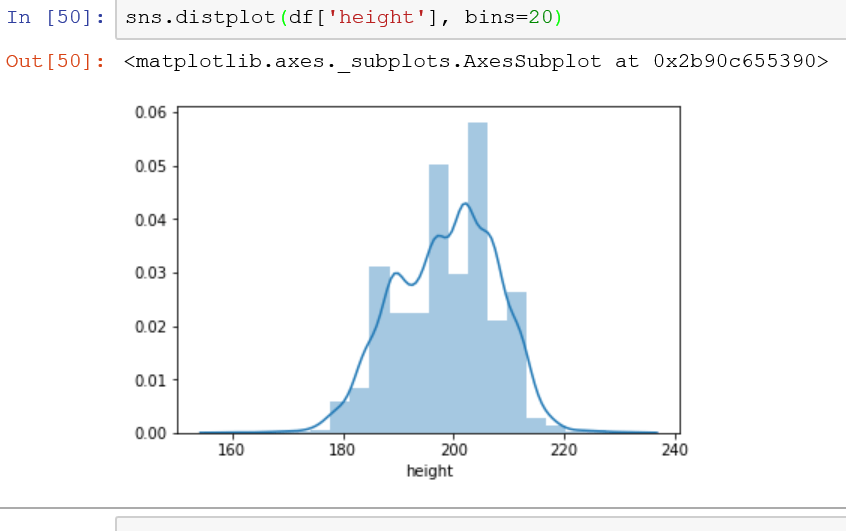• in
• |
• November 11, 2018#### Gaelim Holland

Subscribe
Notify of